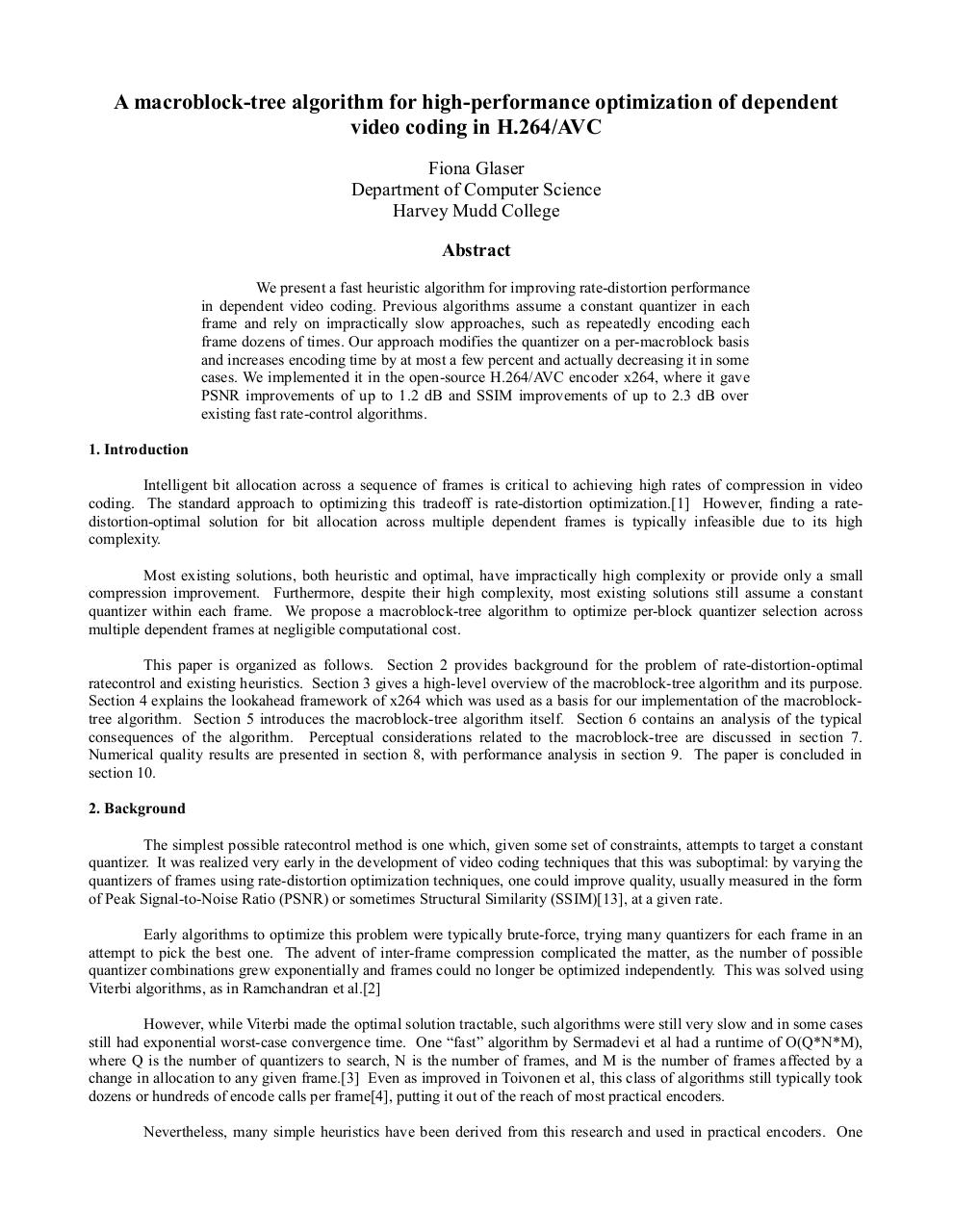# MBtree paper.pdfPage 1 2 3 4 5 6 7 8 9 10 11 12

#### Text preview

A macroblock-tree algorithm for high-performance optimization of dependent
video coding in H.264/AVC
Fiona Glaser
Department of Computer Science
Harvey Mudd College
Abstract
We present a fast heuristic algorithm for improving rate-distortion performance
in dependent video coding. Previous algorithms assume a constant quantizer in each
frame and rely on impractically slow approaches, such as repeatedly encoding each
frame dozens of times. Our approach modifies the quantizer on a per-macroblock basis
and increases encoding time by at most a few percent and actually decreasing it in some
cases. We implemented it in the open-source H.264/AVC encoder x264, where it gave
PSNR improvements of up to 1.2 dB and SSIM improvements of up to 2.3 dB over
existing fast rate-control algorithms.
1. Introduction
Intelligent bit allocation across a sequence of frames is critical to achieving high rates of compression in video
coding. The standard approach to optimizing this tradeoff is rate-distortion optimization. However, finding a ratedistortion-optimal solution for bit allocation across multiple dependent frames is typically infeasible due to its high
complexity.
Most existing solutions, both heuristic and optimal, have impractically high complexity or provide only a small
compression improvement. Furthermore, despite their high complexity, most existing solutions still assume a constant
quantizer within each frame. We propose a macroblock-tree algorithm to optimize per-block quantizer selection across
multiple dependent frames at negligible computational cost.
This paper is organized as follows. Section 2 provides background for the problem of rate-distortion-optimal
ratecontrol and existing heuristics. Section 3 gives a high-level overview of the macroblock-tree algorithm and its purpose.
Section 4 explains the lookahead framework of x264 which was used as a basis for our implementation of the macroblocktree algorithm. Section 5 introduces the macroblock-tree algorithm itself. Section 6 contains an analysis of the typical
consequences of the algorithm. Perceptual considerations related to the macroblock-tree are discussed in section 7.
Numerical quality results are presented in section 8, with performance analysis in section 9. The paper is concluded in
section 10.
2. Background
The simplest possible ratecontrol method is one which, given some set of constraints, attempts to target a constant
quantizer. It was realized very early in the development of video coding techniques that this was suboptimal: by varying the
quantizers of frames using rate-distortion optimization techniques, one could improve quality, usually measured in the form
of Peak Signal-to-Noise Ratio (PSNR) or sometimes Structural Similarity (SSIM), at a given rate.
Early algorithms to optimize this problem were typically brute-force, trying many quantizers for each frame in an
attempt to pick the best one. The advent of inter-frame compression complicated the matter, as the number of possible
quantizer combinations grew exponentially and frames could no longer be optimized independently. This was solved using
Viterbi algorithms, as in Ramchandran et al.
However, while Viterbi made the optimal solution tractable, such algorithms were still very slow and in some cases
still had exponential worst-case convergence time. One “fast” algorithm by Sermadevi et al had a runtime of O(Q*N*M),
where Q is the number of quantizers to search, N is the number of frames, and M is the number of frames affected by a
change in allocation to any given frame. Even as improved in Toivonen et al, this class of algorithms still typically took
dozens or hundreds of encode calls per frame, putting it out of the reach of most practical encoders.
Nevertheless, many simple heuristics have been derived from this research and used in practical encoders. One